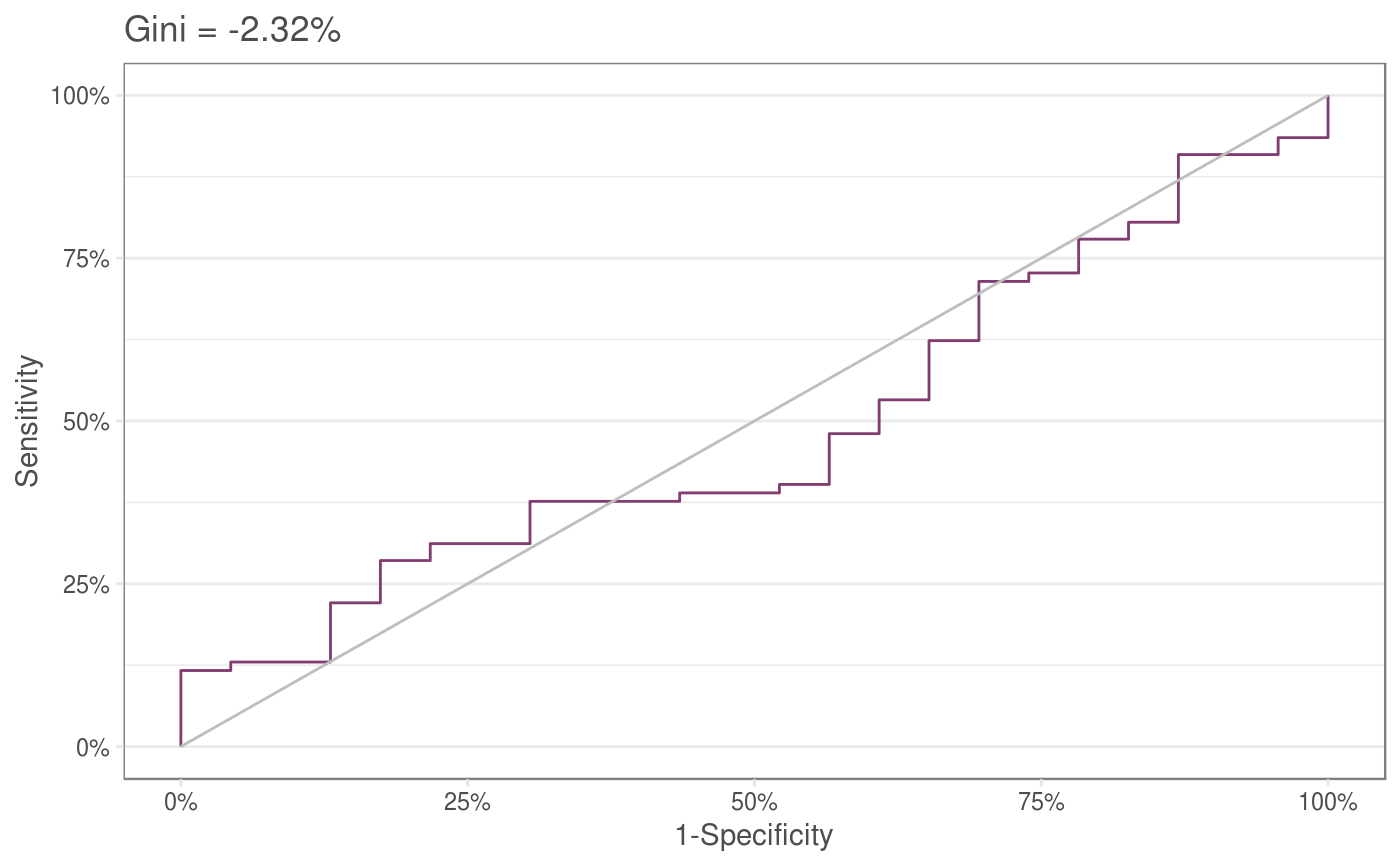This function uses ggplot to produce a themed Receiver Operator Curve and calculates a Gini coefficient based on it.

giniChart(pred, act)

## Arguments

pred Logit/scores/probabilities to be compared against actuals This should be a column containing outcomes in a boolean form either as a factor or number

AUC roc giniCoef
Other creditrisk: giniCoef, logit.odd, logit.prob, odd.logit, odd.prob, prob.logit, prob.odd, scaledScore
  sampledata<- data.frame(val= rnorm(100) , outcome=rbinom(100,1,.8))
giniChart(sampledata$val,sampledata$outcome)